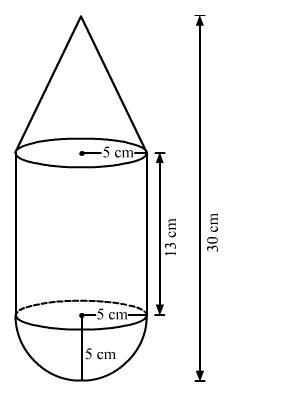# A toy is in the shape of a right circular cylinderQuestion:

A toy is in the shape of a right circular cylinder with a hemisphere on one end and a cone on the other. The radius and height of the cylindrical part are 5 cm and 13 cm respectively. The radii of the hemispherical and conical parts are the same as that of the cylindrical part. Find the surface area of the toy if the total height of the toy is 30 cm.

Solution:

We have the following diagramFor cylindrical part, we have

$h=13 \mathrm{~cm}$

$r=5 \mathrm{~cm}$

Therefore, the curved surface area of the cylinder is given by

$S_{1}=2 \pi r h$

$=2 \times 3.14 \times 5 \times 13$

$=408.2 \mathrm{~cm}^{2}$

For conical part, we have

$h=30-13-5$

$=12 \mathrm{~cm}$

$l=\sqrt{h^{2}+r^{2}}$

$=13 \mathrm{~cm}$

Therefore, the curved surface area of the conical part is

$S_{2}=\pi r l$

$=3.14 \times 5 \times 13$

$=204.1 \mathrm{~cm}^{2}$

For hemisphere, we have

$r=5 \mathrm{~cm}$

Therefore the surface area of the hemisphere is

$S_{3}=2 \pi r^{2}$

$=2 \times 3.14 \times 5^{2}$

$=157 \mathrm{~cm}^{2}$

The total surface area of the toy is

$S=S_{1}+S_{2}+S_{3}$

$=408.2+204.1+157$

$=769.3 \mathrm{~cm}^{2}$

Hence, total surface area of the toy is $S=769.3 \mathrm{~cm}^{2}$